Latest Banking jobs   »   Reasoning Ability Quiz For RBI Grade...

# Reasoning Ability Quiz For RBI Grade B/ ECGC PO/ SIDBI Grade A Prelims 2022- 1st May

Directions (1-5): Study the following information carefully and answer the questions given below:
Seven boxes are placed one above the other. Each box contains different numbers of balls-18, 25, 36, 40, 45, 64 and 70 (but not necessary in the same order).
Box U is below box S. Two boxes are kept between P and the box containing 36 balls which is kept below Box P. T contains double number of balls than box Q. Box R contains 5 ball more than S and is not kept at the top. The number of balls in box V is a square of a number. Only one box is kept between box containing 36 balls and 40 balls. Box S has less number of balls than box P. Five boxes are kept between box containing 64 balls and Box R. Box V is immediately above box T. Box S is not kept immediately above or below box Q. Three boxes are kept between box S and box U. Not more than two boxes are kept above P. Box P contains number of balls which is cube of a number.

Q1. How many boxes are placed above the box which contain 70 balls?
(a) Three
(b) One
(c) Two
(d) More than four
(e) None

Q2. Which of the following box is placed immediate below box Q?
(a) V
(b) The box which contain maximum number of balls
(c) R
(d) The box which contain 36 balls
(e) None of these

Q3. What is the difference between number of balls in box V and box Q?
(a) 20
(b) 15
(c) 7
(d) 10
(e) None of these

Q4. Which of the following is not true about box R?
(a) Box R is placed at bottom
(b) Box T contain 12 balls less than R
(c) Box R contain 45 balls
(d) Only three boxes are placed between R and V
(e) Both (b) and (d)

Q5. Which of the following box contain second lowest number of balls?
(a) V
(b) S
(c) T
(d) Q
(e) None of these

Directions (6-8): Read the following information and answer the questions that follow:
Rajesh walks 10 m south from point A to reach point B. He then takes a right turn and walks 9 m to reach point C. On the other side, Rohit who is north to point B, walks 5 m north from point P to reach point Q. Next ‘Rohit’ turns to his left and walks 4m to reach point R and again takes a left turn and walks 10m to reach point E. Also, Rajesh turned right from point C and reached point D after walking 5 m. Q is 7m either north or south to point B.

Q6. What is the shortest distance between points B and E?
(a) 6 m
(b) 4 m
(c) 8 m
(d) 5 m
(e) Cannot be Determined

Q7. If Rohit walks 4 m east from point E, then he is in which direction with respect to point B?
(a) South
(b) North-west
(c) North-east
(d) North
(e) South-west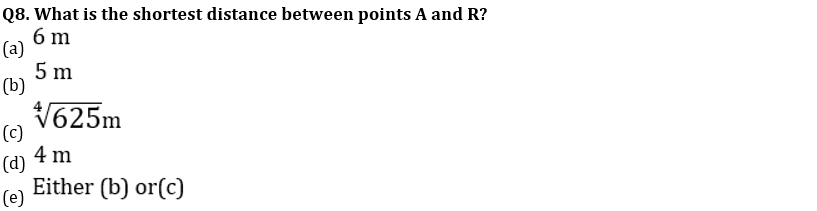Q9. How many pairs of letters are there in the word “PREDICTION” each of which have as many letters between them in the word as they have between them in the English alphabetical series?
(a) One
(b) Two
(c) Three
(d) More than three
(e) None

Q10. In a certain code, ROOMMATE is written as NPPSFUBN. How is SCRABBLE written in the code?
(a) TDSBCCFM
(b) FMCCBSDT
(c) BSDTFMCC
(d) BSDZFMCC
(e) None of these

Directions (11-15): In number arrangement machine when given an input line of numbers, it rearranges them following a particular rule in each step. The following is an illustration of input and rearrangement.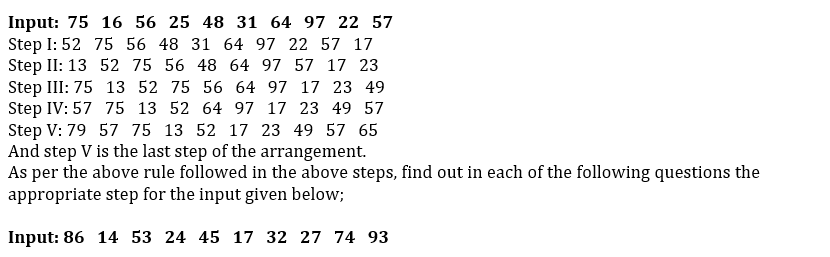Q11. Which of the following is the last step of above input?
(a) III
(b) VI
(c) IV
(d) V
(e) None of these

Q12. Which is the following step?
Step: 54 72 71 86 53 74 93 15 25 33
(a) VI
(b) III
(c) IV
(d) V
(e) There is no such step

Q13. What is 4th element from right end in Step IV?
(a) 15
(b) 71
(c) 86
(d) 93
(e) 54

Q14. Which of the following elements lies exactly between 71 and 32 in step II?
(a) 15
(b) 86
(c) 53
(d) 25
(e) 74

Q15. Which of the following is the fifth step for input?
(a) 39 15 25 33 75 87 35 54 72 71
(b) 39 35 25 33 75 87 54 72 71 15
(c) 39 72 71 15 25 33 35 54 75 87
(d) 71 15 25 33 75 87 39 35 54 72
(e) 39 35 54 72 71 15 25 33 75 87

Solutions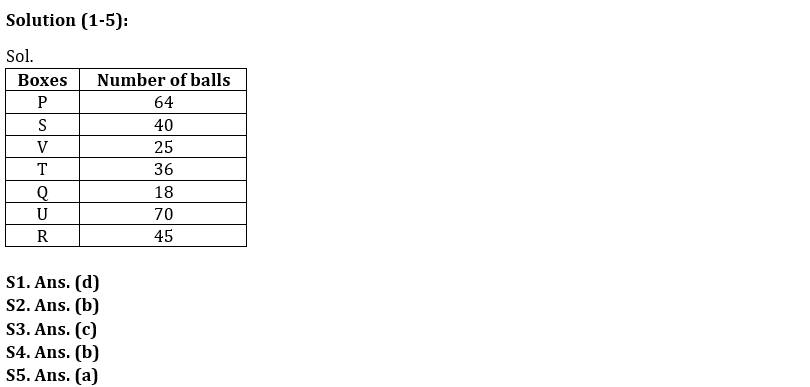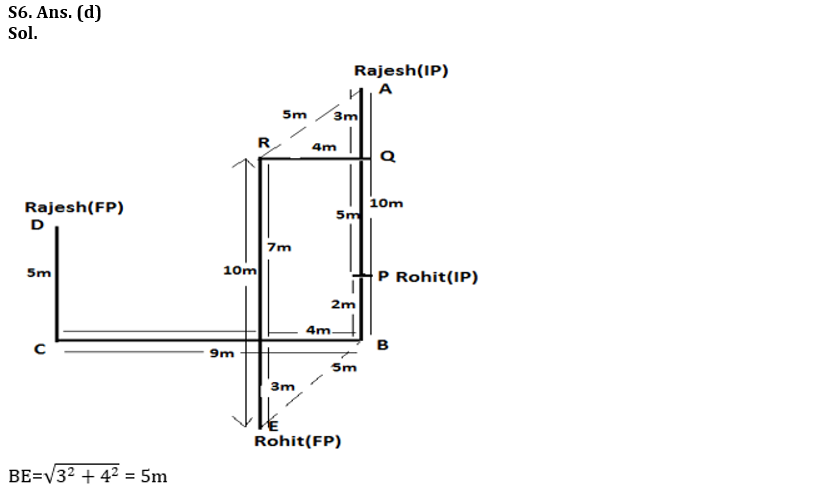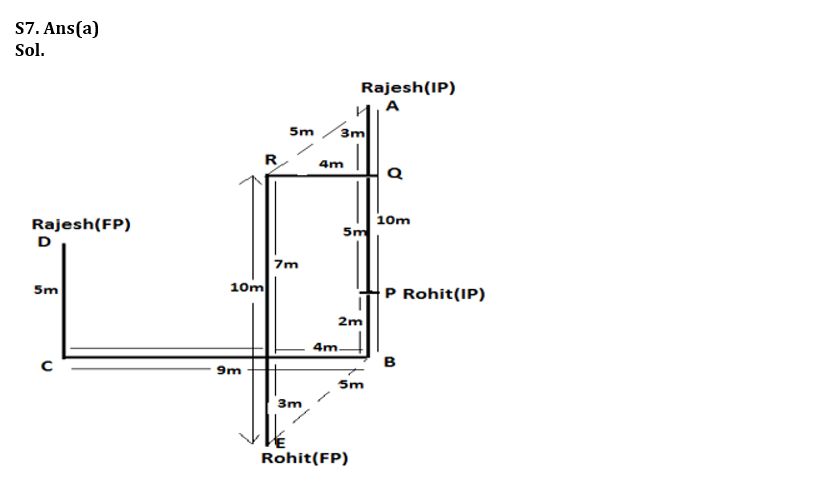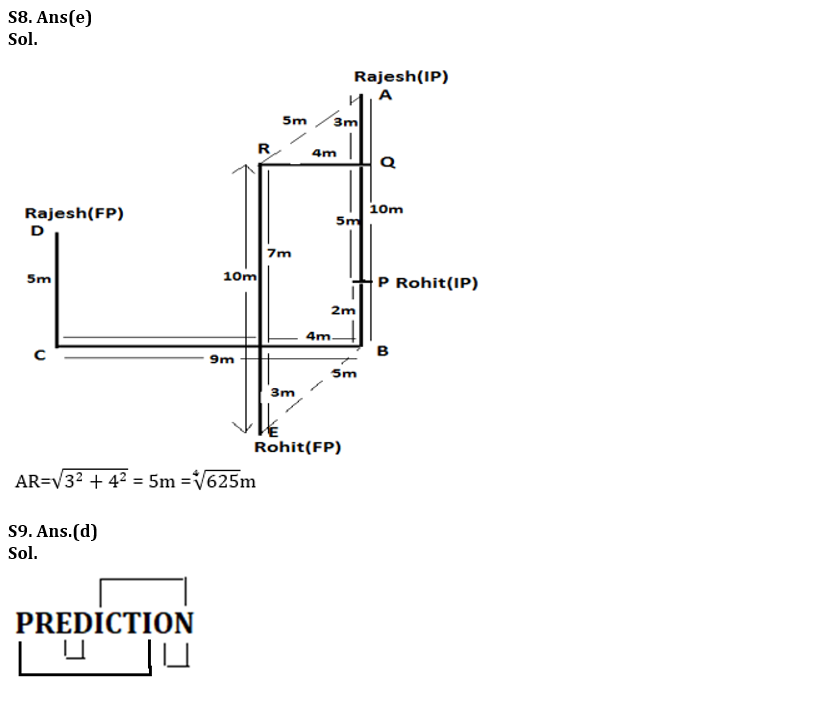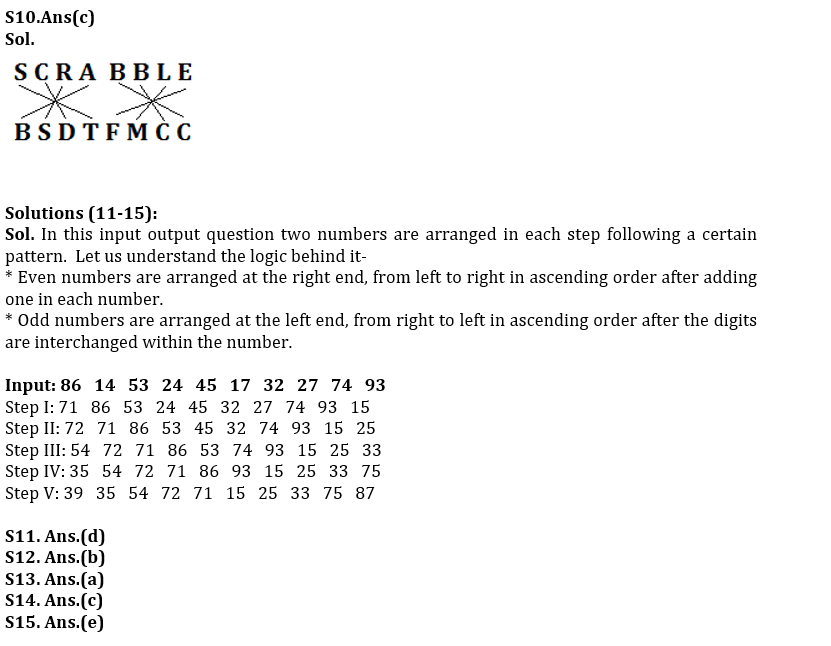#### Congratulations!Union Budget 2023-24: Free PDF